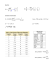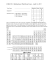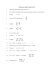# Math 30G – Calculus – Sample Final Exam Fall 2012

## Transcription

Math 30G – Calculus – Sample Final Exam Fall 2012
```Math 30G – Calculus – Sample Final Exam
Fall 2012
The Harvey Mudd College Honor Code applies to this exam. That means
it is a violation of the Honor Code to make this exam accessible in any
format to any person not currently enrolled in this class this semester.
1
16
2
16
3
13
4
20
5
15
6
20
Total
100
1
Problem 1 (2 pts each, 16 pts total). Write T if true, or F if false (with no explanations
needed, no partial credit).
∂ 2f
∂ 2f
+
= 0.
∂x2
∂y 2
(a)
The function f (x, y) = x2 − y 2 + 1 solves the equation
(b)
If ~a · ~b = k~ak k~bk, then ~a and ~b point in the same direction.

(c)

5
The plane containing the point (1, 1, 1) with normal vector −2 has equation
4
5x − 2y + 4z = 0.
(d)
The function f (x, y) = sin xy + cos xy has partial derivative
∂f
= x(cos xy − sin xy).
∂x
(e)
For all continuous functions f ,
Z 2Z y
Z
f (x, y) dx dy =
0
(f)
0
2
Z
0
x
f (x, y) dy dx.
0
Let R be the rectangle in R2 with vertices (2, 1), (2, −1), (−2, −1), and (−2, 1).
Then
ZZ
(x + y 3 ) dA = 0.
R
(g)
P
P∞
n
Let ∞
n=1 an be an absolutely convergent series. Then the series
n=1 (−1) an
must be convergent.
(h)
The integral test can be used to show that the following series converges:
∞
X
n=1
2
n2
.
n3 + 1
Problem 2 (4 pts each, 16 pts total). Short answer. No partial credit, so check your work
carefully. Also, it is not necessary to provide explanations of solutions (for these short answer
problems only).
(a)
d x
7 =
dx
.
(b) Match each quantity on the left below with (i), (ii), or (iii) if possible, or state “no match”.
ln
a
(i) ln a + ln b
b
ln a
ln b
(ii) ln a − ln b
eln a−ln b
(iii)
(ln a)(ln b)
a
b
(c)
If f is continuous
on [a, b], then A(x) =
Z x
d
i.e.,
f (t) dt = f (x).
dx a
Rx
a
f (t) dt is differentiable and A0 (x) = f (x),
This theorem is known as
.
(d) Use the function f (x, y) = xy and the multivariable chain rule to calculate
.
Hint: if we let x(t) = sin t and y(t) = cos t, then we want to find ∂f
∂t
d
dt
[(sin t)cos t ].
(Also, you may receive 1 point of credit for providing the relevant tree diagram.)
3
Problem 3 (13 pts). Let R be the region in the plane bounded by the circle x2 + y 2 = 6 and
below the parabola y = −x2 . Sketch the region R and set up an iterated integral representing
the area of R. (Note: you do not need to evaluate this integral.)
4
Problem 4 (20 pts). Use the indicated technique to compute the following integrals.
Z 1
xe−x dx
(a) Integration by parts:
0
Z
(b) Integration by substitution:
ln(ln x)
dx
x ln x
5
Problem 5 (15 pts). A sphere of radius 3 given by z = f (x, y) is defined implicitly by the
equation:
x2 + y 2 + z 2 = 9.
Find the equation for the tangent plane to the sphere in R3 at the point (1, 2, 2). Box your
6
Problem 6 (20 pts). Let W be the region in the first octant of R3 bounded by x + y + z = 1
and x + y + 2z = 1.
(a) Provide a triple integral representing the volume of W . Write this expression as an iterated
integral (i.e., choose an order of integration and write down the limits of integration).
(b) Evaluate the integral you set up in part (a).
7
```

### Calculus I Final, Sample### Problem Set 3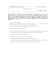### Worksheet 23 11/25/2014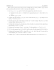### Exam 3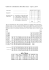### MAT 132 - Calculus II,Practice Problems for Midterm 2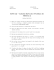### 10.1\\\-10.3 review ans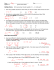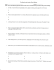### Test4-Review - FIU Faculty Websites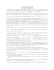### Automata Theory Sample Midterm Exam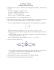### Math 0120 Examination #3 Sample Name (Print)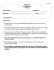### Assignment 2 the 1.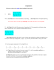### Blank PDF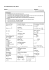### 3w 3 t5+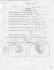### LAST (family) NAME: FIRST (given) NAME: ID # : MATHEMATICS 2C03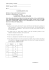### Sample Questions for Final Exam, MATH 2A03/2X03, Spring 2014 Ruipeng Shen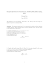### Sample Exam 3 - People Pages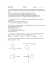### 2 for 1 Dinner Menu - Club Regent Casino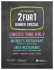### Name _____Mr. Perfect________________________________ Date __F 14_______ n l of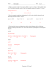### Cover Sheet! EXAM2 PRINT your name legibly on the line below.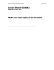### Questions